# Grammar of Graphics with ggplot2

## What is ggplot2?

• ggplot2 is a package inside the mega-package tidyverse.
• Visualizing data using ggplot2 has become the “default” for many R users.
• It is much more elegant and versatile than base R graphing functions.
• But that also comes with the price of more “complex” function calls.

ggplot2 implements the grammar of graphics, a coherent system for describing and building graphs.

## First Steps

• You already have ggplot2 installed in R since it was a part of the tidyverse package.
• So, to “use” ggplot2, you just need to run the following command:
library(ggplot2)
• Now, we’re ready to start graphing!
• Let’s use our first graph to answer a question:
• Do cars with big engines use more fuel than cars with small engines?

### The mpg data frame

• To answer the question above, we will use the mpg dataset that is included in the ggplot2 package.
mpg
## # A tibble: 234 x 11
##    manufacturer model    displ  year   cyl trans   drv     cty   hwy fl    class
##    <chr>        <chr>    <dbl> <int> <int> <chr>   <chr> <int> <int> <chr> <chr>
##  1 audi         a4         1.8  1999     4 auto(l… f        18    29 p     comp…
##  2 audi         a4         1.8  1999     4 manual… f        21    29 p     comp…
##  3 audi         a4         2    2008     4 manual… f        20    31 p     comp…
##  4 audi         a4         2    2008     4 auto(a… f        21    30 p     comp…
##  5 audi         a4         2.8  1999     6 auto(l… f        16    26 p     comp…
##  6 audi         a4         2.8  1999     6 manual… f        18    26 p     comp…
##  7 audi         a4         3.1  2008     6 auto(a… f        18    27 p     comp…
##  8 audi         a4 quat…   1.8  1999     4 manual… 4        18    26 p     comp…
##  9 audi         a4 quat…   1.8  1999     4 auto(l… 4        16    25 p     comp…
## 10 audi         a4 quat…   2    2008     4 manual… 4        20    28 p     comp…
## # … with 224 more rows
• The variables(columns) we care about are:
• displ: a car’s engine size, in litres.
• hwy: a car’s fuel efficiency on the highway, in miles per gallon (mpg).

### Our first ggplot2 plot

ggplot(data = mpg) +
geom_point(mapping = aes(x = displ, y = hwy))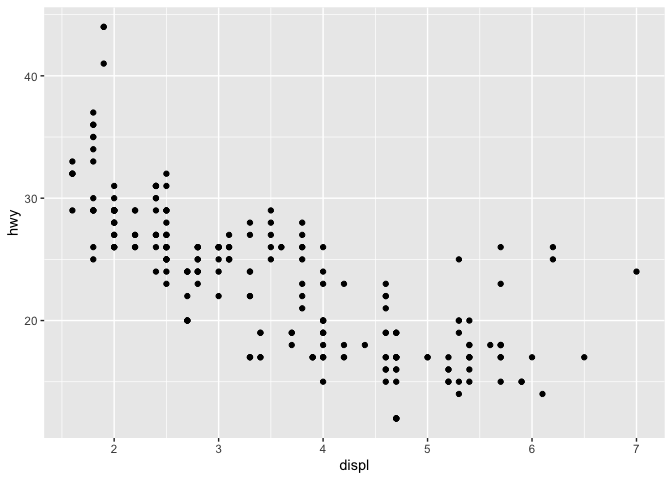• The above code graphs a scatterplot with displ on the $$x$$-axis and hwy on the $$y$$-axis.
• Now, we will try to interprete the grammar of the code segment above:
• With ggplot2, you begin a plot with the function ggplot().
• ggplot() creates a coordinate system that you can add layers to.
• The first argument of ggplot() is the dataset to use in the graph.
• So ggplot(data = mpg) creates an empty graph (see below)
ggplot(data = mpg)• That is NOT interesting at all, right?! 👎👎👎
• To make the plot interesting, we need to add layers!
• You can add one or multiple layers to the plot.
• geom_point() function add a layer of points (as the name suggested!).

### A graphing template

• The following code segment is a reusable template for making graphs with ggplot2.
ggplot(data = <DATA>) +
<GEOM_FUNCTION>(mapping = aes(<MAPPINGS>))
• To make a graph, replace the bracketed sections in the code below with a dataset, a geom function, or a collection of mappings.

### The geom functions

• There are many geom() functions in ggplot2: geom_boxplot(), geom_line(), etc.
• Each geom function takes a mapping argument. This defines how variables in your dataset are mapped to visual properties.
• The mapping argument is always paired with aes().
• The x and y arguments of aes() specify which variables to map to the x and y axes.
• ggplot2 looks for the mapped variables in the data argument, in this case, mpg.

### Examples

• Example 1: make a scatterplot of hwy vs cyl.
• Example 2: make a scatterplot of class vs drv.

Notes: To see my code, take a look at the lecture video! 😉

## Aesthetic Mappings

• Aesthetic Mapping uses the aes() function as described above.
• An aesthetic is a visual property of the objects in your plot.
• Aesthetics include things like the size, the shape, or the color of your points.

### Add color to your plot

ggplot(data = mpg) +
geom_point(mapping = aes(x = displ, y = hwy, color = class))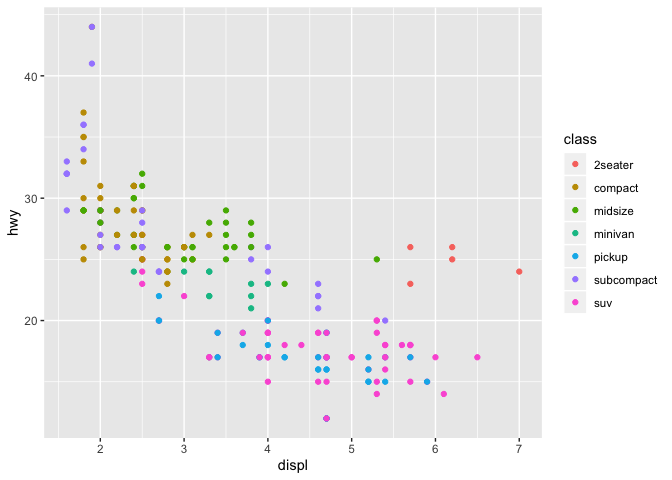• With aes(..., color = class), ggplot2 will automatically assign a unique level of the aesthetic (here a unique color) to each unique value of the variable (here class), a process known as scaling.
• ggplot2 will also add a legend that explains which levels correspond to which values.

• Now, what’s if you don’t want to color the points by class, you just want all the points to be blue!
• Let’s try changing class to "blue".
ggplot(data = mpg) +
geom_point(mapping = aes(x = displ, y = hwy, color = "blue"))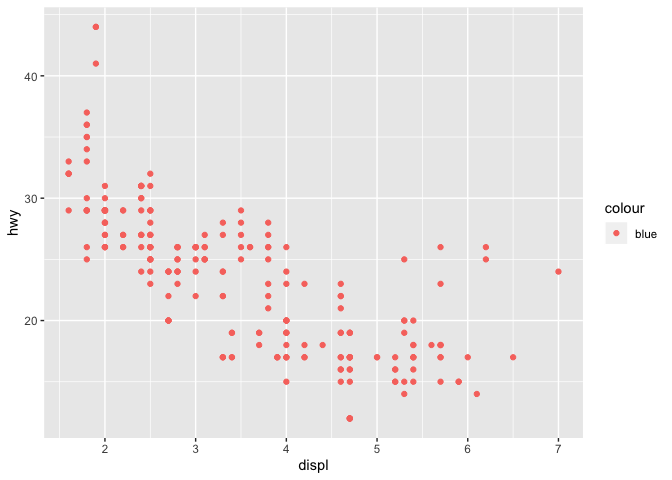• Hmm! 😕 That did not seem to work!
• Try something slightly different,
ggplot(data = mpg) +
geom_point(mapping = aes(x = displ, y = hwy), color = "blue")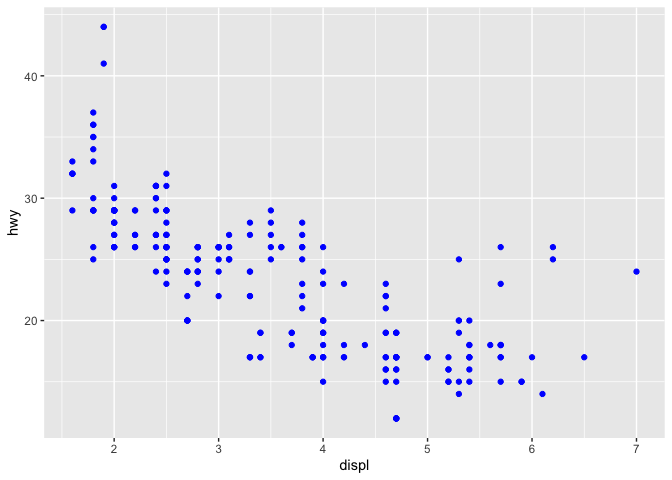• It works! 👏👏👏 So, what is the difference?

### Change shape of the points

ggplot(data = mpg) +
geom_point(mapping = aes(x = displ, y = hwy, shape = class))
## Warning: The shape palette can deal with a maximum of 6 discrete values because
## more than 6 becomes difficult to discriminate; you have 7. Consider
## specifying shapes manually if you must have them.
## Warning: Removed 62 rows containing missing values (geom_point).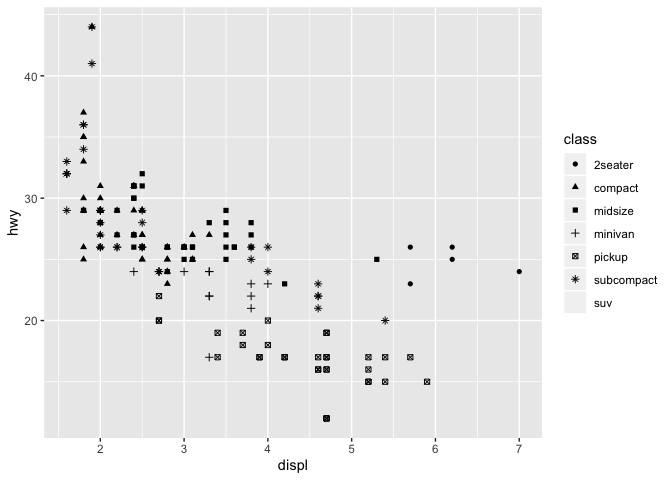• Notice how the SUVs are not shown on the plot? What is the reason for that?

### Change the transparency of the points

ggplot(data = mpg) +
geom_point(mapping = aes(x = displ, y = hwy, alpha = class))
## Warning: Using alpha for a discrete variable is not advised.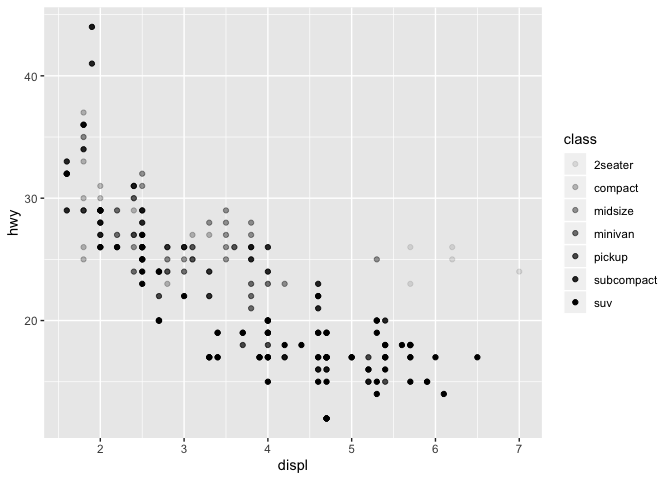• See the warning message? What does that mean?
• Let’s try setting alpha to a continuous variable.
ggplot(data = mpg) +
geom_point(mapping = aes(x = displ, y = hwy, alpha = cty))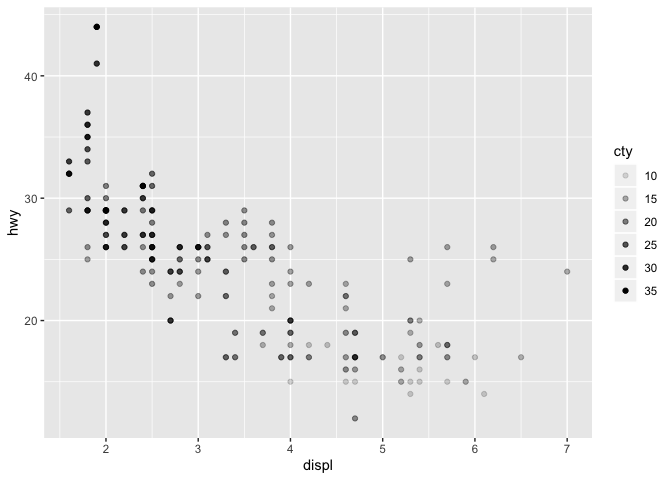• That’s a bit difficult to see. Let’s change the color.
ggplot(data = mpg) +
geom_point(mapping = aes(x = displ, y = hwy, alpha = cty), color = "red")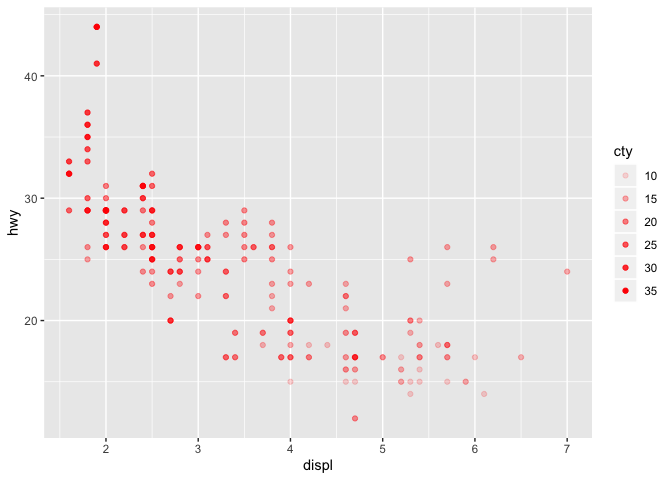### Examples

• Example 1: What happens if you map the same variable to multiple aesthetics?
• Example 2: What does the stroke aesthetic do? What shapes does it work with?
• Example 3: What happens if you map an aesthetic to something other than a variable name, like aes(colour = displ < 5)? Note, you’ll also need to specify x and y.

## Common Problems

• One common problem when creating ggplot2 graphics is to put the + in the wrong place:
• It has to come at the end of the line, not the start.
ggplot(data = mpg)+ geom_point(mapping = aes(x = displ, y = hwy))
## Error: Cannot use +.gg() with a single argument. Did you accidentally put + on a new line?